# SSC CGL 2018 Practice Test Papers | Quantitative Aptitude (Day-45)

Dear Aspirants, Here we have given the Important SSC CGL Exam 2018 Practice Test Papers. Candidates those who are preparing for SSC CGL 2018 can practice these questions to get more confidence to Crack SSC CGL 2018 Examination.

[WpProQuiz 3439]

Click “Start Quiz” to attend these Questions and view Explanation

The table given below shows a survey carried out at a railway station for the arrivals/ departure of trains for the month of January 2010.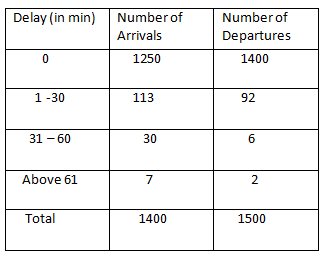1) How many trains are arrived late to the station?

a) 140

b) 37

c) 150

d) 113

2) What is the total number of late departures from the station?

a) 100

b) 150

c) 92

d) 94

3) The number of trains arrived about 31 – 60 minutes late is what percentage of total number of late departures from the station?

a) 50%

b) 30%

c) 25%

d) 20%

4) If the punctuality of Railways is defined as the number of occasions on which trains arrived or departed on time as a percentage of total number arrivals and departures from the station, then the punctuality for the month under observation is

a) 91.7

b) 91.4

c) 92.3

d) 93.3

5) If the number of total arrivals to the station is increased by 100 and there is no change in trains arrived late in (0-30) and (31-60) minutes category. If the number of trains arrived on time is increased by 4% what is the number of trains arrived late above 60 minutes?

a) 75

b) 57

c) 67

d) 50

6) The angle of elevation of the top of the tree from a point which is 45√3 m from the base of the tree is 30o. Find the height of the tower.

a) 45 m

b) 45√3 m

c) 135 m

d) 120 m

7) In a right angled triangle PQR, right angled at Q, if PQ=3√6 and PR-QR= 2, what is the value of sin R+ cos R.

a) (1+2√6)/7

b) (5+2√6)/10

c) (7+2√6)/7

d) (8+3√6)/10

8) Find the equation of the line which make x and y intercepts in the ratio 1: 2 and passes through points (2,4)

a) x+2y=10

b) 2x-y=8

c) 2x+y=10

d) 2x+y=8

9) Area of triangle by straight line ax+by+c=0, a0, b0 with coordinate axes is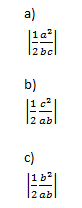d) None of the above

10) The equation ax2+bx+c=0 cannot be a quadratic equation if

a) b = 0

b) c = 0

c) a = 0

d) Both a and b

Direction (1-5) :

Number of trains arrived late = 113 + 30 + 7 = 150

= 92 + 6 + 2 = 100

Number of trains arrived (31 – 60) minutes late = 30

Total number of late departures = 92 + 6 + 2 = 100

Required Percentage = 30/100 x100 = 30%

Number of occasions on which trains arrived or departed on time = 1250 + 1400 = 2650

Total number arrivals and departures from the station = 1400 + 1500 = 2900

Required percentage = 2650/2900 x100 = 91.4%

Since there is no change in trains arrived late in (0-30) and (31-60) minutes category, changes will be in trains arrived on time and arrived Over 60 min late.

Number of total arrivals to the station = 1400 + 100 = 1500

Trains arrived on time = 1250×104/100 = 1300

The number of trains arrived late above 60 minutes = 7+100 – 50 = 57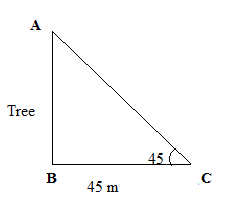In Δ ABC,

tan 30= AB/BC( tan θ= Perpendicular/base)

1/√3= AB/45√3

AB=45m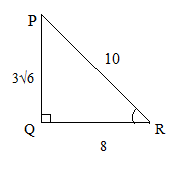PQ=3√6

PR-QR=2 —– (i)

PR2=PQ2+QR2

PQ2=PR2-QR2

(3√6)2=(PR+QR)(PR-QR)

54=(PR+QR)(3)

(PR+QR)=18 —— (ii)

On solving(i) and(ii)

PR=10 and QR= 8

Cos R= 8/10=4/5

Sin R=3√6/10

Sin R+ Cos R= (8+3√6)/10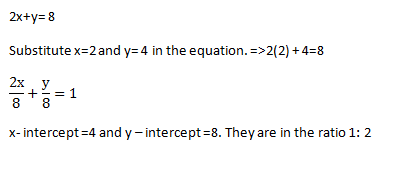2x+y= 8

Substitute x=2 and y= 4 in the equation. =>2(2)+4=8

x- intercept =4 and y – intercept =8. They are in the ratio 1: 2

A Quadratic equation is an equation in which the highest power of an unknown quantity is a square that can be written as ax2+bx+c=0.

Therefore coefficient of x2 cannot be zero.